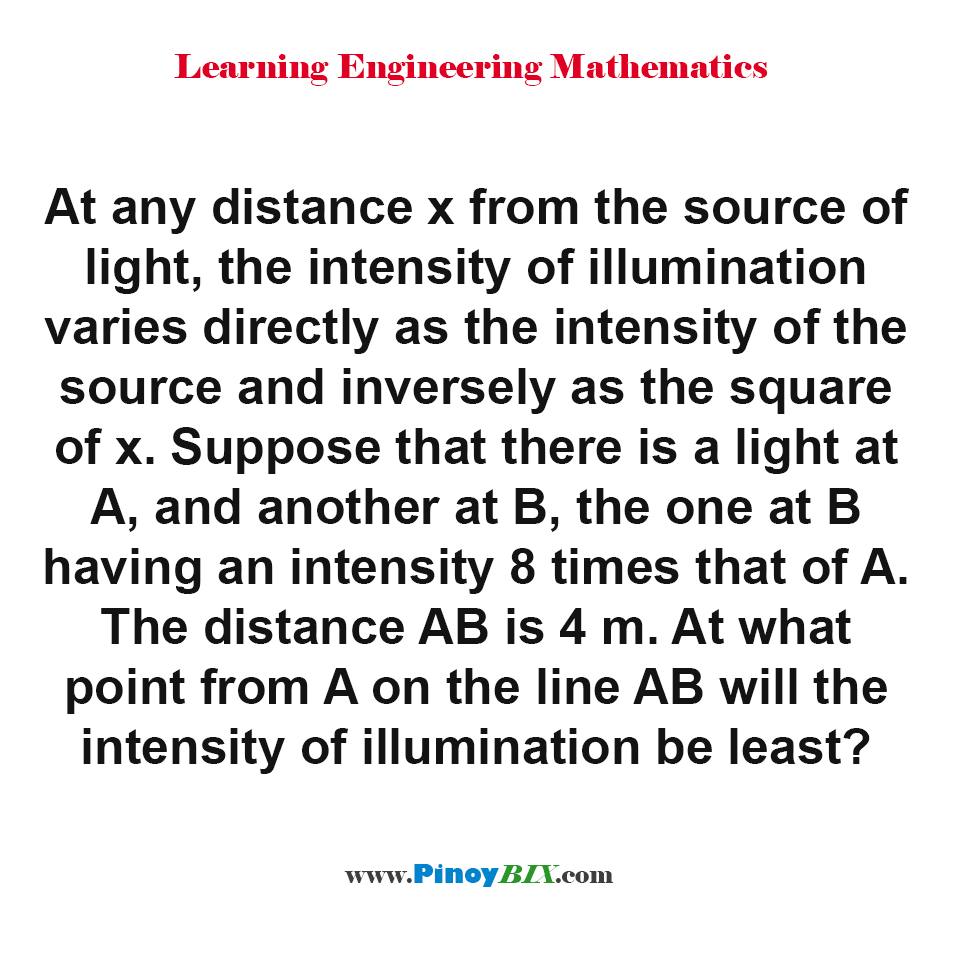# Solution: At what point from A on the line AB will the intensity of illumination be least?

(Last Updated On: January 21, 2020)#### Problem Statement: EE Board October 1993

At any distance x from the source of light, the intensity of illumination varies directly as the intensity of the source and inversely as the square of x. Suppose that there is a light at A, and another at B, the one at B having an intensity 8 times that of A. The distance AB is 4 m. At what point from A on the line AB will the intensity of illumination be least?

• A. 2.15 m
• B. 1.33 m
• C. 1.50 m
• D. 1.92 m

At 1.50 m from A on the line AB will the intensity of illumination be least

#### Latest Problem Solving in Differential Calculus (MAXIMA-MINIMA & TIME RATES)

More Questions in: Differential Calculus (MAXIMA-MINIMA & TIME RATES)